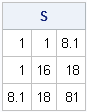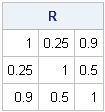Both covariance matrices and correlation matrices are used frequently in multivariate statistics. You can easily compute covariance and correlation matrices from data by using SAS software. However, sometimes you are given a covariance matrix, but your numerical technique requires a correlation matrix. Other times you are given a correlation matrix, but you really need the covariance. This article shows how convert from one to the other.

### Converting a Correlation Matrix to a Covariance Matrix

In order to convert a p x p correlation matrix to a covariance matrix, you need the variances (or standard deviations) of the p variables. Recall that the ijth element of the correlation matrix is related to the corresponding element of the covariance matrix by the formula Rij = Sij / mij where mij is the product of the standard deviations of the ith and jth variables. You can rescale the correlation matrix by pre- and post-multiplying by a diagonal matrix that contains the standard deviations:
```proc iml; /** convert correlation matrix to covariance matrix **/ R = {1.00 0.25 0.90, 0.25 1.00 0.50, 0.90 0.50 1.00 };   /** standard deviations of each variable **/ c = {1 4 9}; D = diag(c);   S = D*R*D; /** covariance matrix **/ print S;```Of course, pre-multiplying by a diagonal matrix (that is D*R) is the same as multiplying each column by the corresponding standard deviation. Similarly, post-multiplying by a diagonal matrix (that is R*D) is the same as multiplying each row by the corresponding standard deviation. This means that you can also convert the correlation matrix by using the following (more efficient!) SAS/IML shorthand operations:
`S2 = c#R#c`;`
A third computation is to implement the formula that relates the correlation and covariance matrices: Rij = Sij / mij. For example:
`S3 = (c`*c) #R;`
Which method is most efficient? I encourage you to time the performance of each method on large matrices in order to find out.

### Converting a Covariance Matrix to a Correlation Matrix

You can use similar operations to convert a covariance matrix to a correlation matrix. First, use the DIAG function to extract the variances from the diagonal elements of the covariance matrix. Then invert the matrix to form the diagonal matrix with diagonal elements that are the reciprocals of the standard deviations.
```/** convert covariance matrix to correlation matrix **/ S = {1.0 1.0 8.1, 1.0 16.0 18.0, 8.1 18.0 81.0 };   /** standard deviations of each variable **/ D = sqrt(diag(S)); DInv = inv(D); R = DInv * S * Dinv; /** correlation matrix **/ print R;```Again, you can use SAS/IML shorthand operations to scale the covariance matrix without having to invert a diagonal matrix. This results in code that is much more efficient:
```D = sqrt(vecdiag(S)); R2 = S / D` / D; /** divide columns, then divide rows **/```
The third method, which uses the formula Rij = Sij / mij, is left as an exercise.

ShareDistinguished Researcher in Computational Statistics

Rick Wicklin, PhD, is a distinguished researcher in computational statistics at SAS and is a principal developer of SAS/IML software. His areas of expertise include computational statistics, simulation, statistical graphics, and modern methods in statistical data analysis. Rick is author of the books Statistical Programming with SAS/IML Software and Simulating Data with SAS.

1.2.